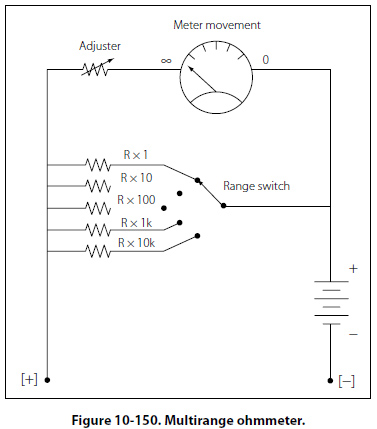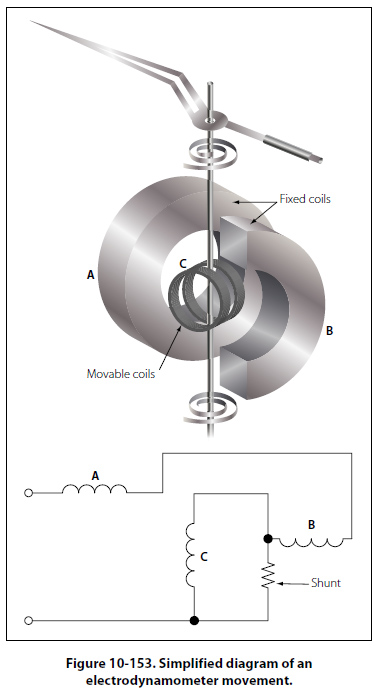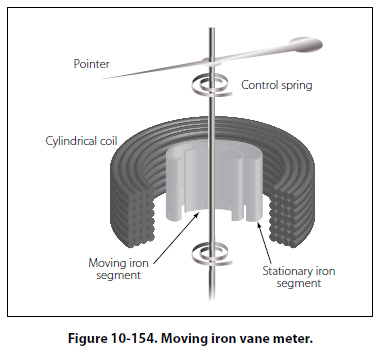AC Measuring Instruments A DC meter, such as an ammeter, connected in an AC circuit will indicate zero, because the meter movements used in a d’Arsonval type movement is restricted to direct current. Since the field of a permanent magnet in the d’Arsonval type meter remains constant and in the same direction at all times, the moving coil follows the polarity of the current. The coil attempts to move in one direction during half of the AC cycle and in the reverse direction during the other half when the current reverses. The current reverses direction too rapidly for the coil to follow, causing the coil to assume an average position. Since the current is equal and opposite during each half of the AC cycle, the direct current meter indicates zero, which is the average value. Thus, a meter with a permanent magnet cannot be used to measure alternating voltage and current. For AC measurements of current and voltage, additional circuitry is required. The additional circuitry has a rectifier, which converts AC to DC. There are two basic types of rectifiers: One is the half-wave rectifier and the other is the full-wave rectifier. Both of these are depicted in block diagram form in Figure 10-152.Figure 10-152 also shows a simplified block diagram of an AC meter. In this depiction, the full-wave rectifier precedes the meter movement. The movement responds to the average value of the pulsating DC. The scale can then be calibrated to show anything the designer wants. In most cases, it will be root mean square (RMS) value or peak value. Electrodynamometer Meter Movement The electrodynamometer can be used to measure alternating or direct voltage and current. It operates on the same principles as the permanent magnet moving coil meter, except that the permanent magnet is replaced by an air core electromagnet. The field of the electrodynamometer is developed by the same current that flows through the moving coil. [Figure 10-153]Because this movement contains no iron, the electrodynamometer can be used as a movement for both AC and DC instruments. Alternating current can be measured by connecting the stationary and moving coils in series. Whenever the current in the moving coil reverses, the magnetic field produced by the stationary coil reverses. Regardless of the direction of the current, the needle will move in a clockwise direction. However, for either voltmeter or ammeter applications, the electrodynamometer is too expensive to economically compete with the d’Arsonval type movement. Moving Iron Vane Meter The moving iron vane meter is another basic type of meter. It can be used to measure either AC or DC. Unlike the d’Arsonval meter, which employs permanent magnets, it depends on induced magnetism for its operation. It utilizes the principle of repulsion between two concentric iron vanes, one fixed and one movable, placed inside a solenoid. A pointer is attached to the movable vane. [Figure 10-154]When current flows through the coil, the two iron vanes become magnetized with north poles at their upper ends and south poles at their lower ends for one direction of current through the coil. Because like poles repel, the unbalanced component of force, tangent to the movable element, causes it to turn against the force exerted by the springs. The movable vane is rectangular in shape and the fixed vane is tapered. This design permits the use of a relatively uniform scale. When no current flows through the coil, the movable vane is positioned so that it is opposite the larger portion of the tapered fixed vane, and the scale reading is zero. The amount of magnetization of the vanes depends on the strength of the field, which, in turn, depends on the amount of current flowing through the coil. The force of repulsion is greater opposite the larger end of the fixed vane than it is nearer the smaller end. Therefore, the movable vane moves toward the smaller end through an angle that is proportional to the magnitude of the coil current. The movement ceases when the force of repulsion is balanced by the restraining force of the spring. Because the repulsion is always in the same direction (toward the smaller end of the fixed vane), regardless of the direction of current flow through the coil, the moving iron vane instrument operates on either DC or AC circuits. Mechanical damping in this type of instrument can be obtained by the use of an aluminum vane attached to the shaft so that, as the shaft moves, the vane moves in a restricted air space. When the moving iron vane meter is used as an ammeter, the coil is wound with relatively few turns of large wire in order to carry the rated current. When the moving iron vane meter is used as a voltmeter, the solenoid is wound with many turns of small wire. Portable voltmeters are made with self-contained series resistance for ranges up to 750 volts. Higher ranges are obtained by the use of additional external multipliers. The moving iron vane instrument may be used to measure direct current but has an error due to residual magnetism in the vanes. Reversing the meter connections and averaging the readings may minimize the error. When used on AC circuits, the instrument has an accuracy of 0.5 percent. Because of its simplicity, relatively low cost, and the fact that no current is conducted to the moving element, this type of movement is used extensively to measure current and voltage in AC power circuits. However, because the reluctance of the magnetic circuit is high, the moving iron vane meter requires much more power to produce full-scale deflection than is required by a d’Arsonval meter of the same range. Therefore, the moving iron vane meter is seldom used in high resistance low power circuits.
 ©AvStop Online Magazine                                                                                                                                                      Contact Us              Return To Books